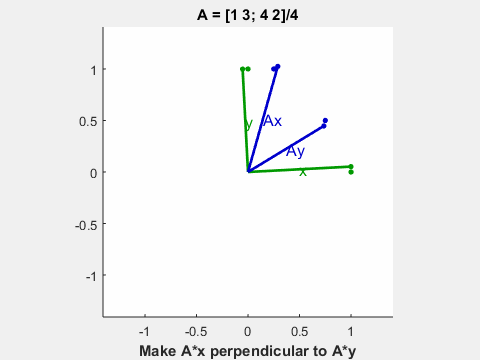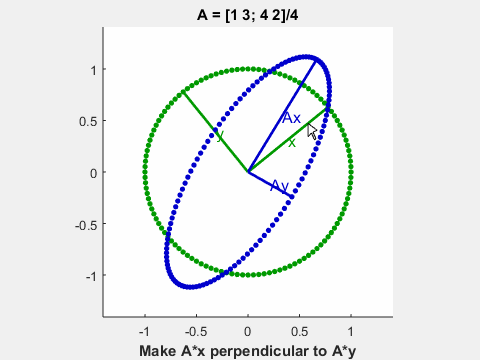# Bug Report Revives Interest in SVD Option of “Eigshow”

A few days ago we received email from Mike Hennessey, a mechanical engineering professor at the University of St. Thomas in St. Paul, Minnesota. He has been reading my book “Numerical Computing with MATLAB” very carefully. Chapter 7 is about “Eigenvalues and Singular Values” and section 10.3 is about one of my all-time favorite MATLAB demos, eigshow. Mike discovered an error in my description of the svd option of eigshow that has gone unnoticed in the over ten years that the book has been available from both the MathWorks web site and SIAM.

### Contents#### Eigshow

The program eigshow has been in the MATLAB demos directory for many years. I wrote a three-part series of posts about eigshow in this blog three years ago, but I’m happy to write another post now.

The svd option of eigshow invites you to use your mouse to move the green vector x and make A*x perpendicular to A*y. The animated gif above simulates that motion. The following figure is the desired final result.As you move x, the vector y follows along, staying perpendicular to x. The two trace out the green unit circle. The default matrix A is shown in the title. (If you run your own eigshow you can change the matrix by editing the text box in the title.)

   A = [1 3; 4 2]/4

A =
0.2500    0.7500
1.0000    0.5000


The blue vectors Ax and Ay are the images of x and y under the multiplicative mapping induced by A. As you move x and y around the unit circle Ax and Ay sweep out the blue ellipse. And when you stop with Ax perpendicular to Ay, they turn out to be the major and minor axes of the ellipse.

#### NCM

Numerical Computing with MATLAB, which is known to its friends as simply NCM, was published over 10 years ago, in 2004. An electronic edition is available from MathWorks and a print edition from SIAM. In chapter 7, the singular value decomposition, the SVD, of a real matrix, $A$, is defined as the product

$$A = U \Sigma V^T$$

In the simplest case where we assume $A$ is square, $U$ and $V$ are orthogonal and $\Sigma$ is diagonal.

This is illustrated by figure 10.3 in NCM, which is the same as the final figure here. It shows the action with the svd option on the default matrix. The explanation is given by the first sentence on page 7.

The vectors x and y are the columns of U in the SVD, the vectors
Ax and Ay are multiples of the columns of V, and the lengths of
the axes are the singular values.

Sounds OK, right? This is the sentence that Mike Hennessey questioned. Now that I call your attention to it, you should be able to spot the error right away. I’ll tell you what it is at the end of this post.

#### help eigshow

Here is the relevant paragraph from the help entry for eigshow . It does not get into the same trouble as NCM.

In svd mode, the mouse moves two perpendicular unit vectors, x and y.
The resulting A*x and A*y are plotted. When A*x is perpendicular to
A*y, then x and y are right singular vectors, A*x and A*y are
multiples of left singular vectors, and the lengths of A*x and A*y
are the corresponding singular values.

#### SVD

Here’s how I often think about the SVD. Take the definition,

$$A = U \Sigma V^T$$

Multiply both sides on the right by $V$.

$$A V = U \Sigma$$

The diagonal matrix $\Sigma$ is on the right so that the singular values can multiply the columns of $U$. When we write this out column by column, we have

$$A v_j = \sigma_j u_j, \ \ j = 1,\ …, n$$

A little bit more manipulation leads to

$$A^T u_j = \sigma_j v_j, \ \ j = 1,\ …, n$$

#### Example

Let’s see how this works out with the default 2-by-2 matrix.

   A

A =
0.2500    0.7500
1.0000    0.5000


Compute the SVD.

   [U,S,V] = svd(A)

U =
-0.5257   -0.8507
-0.8507    0.5257
S =
1.2792         0
0    0.4886
V =
-0.7678    0.6407
-0.6407   -0.7678


The singular values are on the diagonal of S.

   sigma = diag(S)

sigma =
1.2792
0.4886


These are the lengths of the two blue vectors.

#### Dominant singular vector

It turns out that the components of $v_1$, the right singular vector corresponding to the largest singular value of an (irreducible) matrix with nonnegative elements all have the same sign. They could be all positive or all negative — take your pick. MATLAB happens to always pick negative. So, both components of V(:,1) are negative. But we stopped eigshow with a positive x. So we flip the sign of V(:,1).

   x = -V(:,1)
y = V(:,2)

x =
0.7678
0.6407
y =
0.6407
-0.7678


These two look like the two green vectors in the final figure. And these look like the two blue vectors.

   Ax = A*x
Ay = A*y

Ax =
0.6725
1.0881
Ay =
-0.4156
0.2569


Now check that the SVD relationship works.

   AV = A*V
USigma = U*S

AV =
-0.6725   -0.4156
-1.0881    0.2569
USigma =
-0.6725   -0.4156
-1.0881    0.2569


#### The Correction

What was the error in NCM that Mike Hennessey reported and that prompted me to write this post? The matrices U and V should be switched. The sentence should read

The vectors x and y are the columns of V in the SVD, the vectors
Ax and Ay are multiples of the columns of U, and the lengths of
the axes are the singular values.

Every time I work with the SVD I have to think carefully about U and V. Which is which? Maybe writing this post will help.

#### Reference

Cleve Moler, Numerical Computering With MATLAB, 2004.

MathWorks, <https://www.mathworks.com/moler/chapters.html>,

Published with MATLAB® R2016a

|